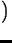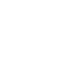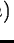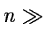Next: Time domain analysis Up: Basic principles of time Previous: Corrections to the probability

## Power of test statistics

The question which method of the detection of features in the test statistic is the most sensitive, is of considerable importance for all practical TSA applications. It directly translates into the comparison of the power of different statistics. Let Y be a signal of some physical meaning, i.e. different from the pure noise. A powerof the test statistic is the probability that Ho is accepted for the given Y:. In other words, this is the probability of the false rejection of the no-noise signal Y as pure noise. Generally, the relative power of various statistics depends on the type of the signal Y. An important practical consequence of this fact is that there is no such thing as the universally best method for time series analysis. A method that is good for one type of signals may be poor for another one. An extensive list of signal types and recommended methods is clearly out of scope of the present manual. However, in order to guide the user's intuition we quote below the results of a comparison of the powers of various test statistics for two types of oscillations, both of small amplitude and observed at more or less even intervals (Schwarzenberg-Czerny, 1989):

Let us first consider a sinusoidal oscillation with an amplitude not exceeding the noise. Then, all statistics based on models with 3 parameters have similar values of the test power: power spectrum, Scargle statistics,fit of a sinusoid, ORT(1), AOV(3) and corrected PDM(3) statistics. (Please note that we depart here from the conventional notation by indicating in brackets the number nm of the degrees of freedom of the model - e.g. the number of series terms or phase bins - instead of the number of the residual degrees of freedom nr.) Statistics with more than 3 parameters, e.g. ORT(n/2+1), AOV(n), PDM(n) andwith n>3 and an extended Fourier series have less power. Our final choice is guided by the availability of the analytical probability distribution of the test statistics. Summing up, we recommend tu use for the detection of sinusoidal and other smooth oscillations of small amplitude the statistics with a coarse phase resolution, e.g. ORT(1), Scargle and AOV(3), .

For a narrow gaussian pulse or eclipse of width w repeating with period P the most powerful statistics are these with the matching resolution: ORT(P/2w), AOV(P/w) andP/w). Power spectrum, Scargle, ORT(1), AOV(3),, ORT(n/2), AOV(n) and,all have less power. Note the equivalence of theand Scargle's statistic (Lomb, 1976, Scargle, 1982) and the near-equivalence of the power spectrum and Scargle's statistics in the case of nearly uniformly sampled observations. Considering both test power and computational convenience we recommend for signals with sharp features, e.g. narrow pulses or eclipses, to use the ORT and AOV with the resolution matched to the width of these features.Next: Time domain analysis Up: Basic principles of time Previous: Corrections to the probability
Petra Nass
1999-06-15